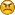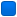###Author Topic: Reverse Polish Notation  (Read 701 times)

####HellOfMice##### Reverse Polish Notation
« on: November 23, 2022, 10:28:49 AM »
I am trying to write a kind of interpretor.
Into I have a function to build RPN expressions
When '()' are used no problem.
When '()' are not used I don't know how to do with operators priorities.
Can some one help me?

Merci

PS: It is a 7Zip file joint not a TRUE ZIP
Kenavo

####John Z

• Member
•• Posts: 594##### Re: Reverse Polish Notation
« Reply #1 on: November 23, 2022, 11:08:43 AM »
Hi Hello....,

When using RPN notation the operator precedence is always just left to right.  The trick, if any, is that when converting from Polish AOS Notation thoughts into RPN one understands and applies the standard mathematical precedence when constructing the RPN notation which is where the () mostly comes in.

example:

if doing 7=3*2+1,  RPN is 7=3 2 * 1 +  if doing 8= (3 +1)*2 , RPN is 8=3 1 + 2 * but if  5= 3+1*2 then
RPN is 5= 1 2 * 3 +  or RPN is 5 = 3 1 2 * +

There are some great  HP calculator emulators free for windows, iOS and Android which can use RPN
HP48x is my favorite

Hope this help,

John Z
« Last Edit: November 23, 2022, 02:36:48 PM by John Z »

####HellOfMice##### Re: Reverse Polish Notation
« Reply #2 on: November 23, 2022, 11:55:54 AM »
Thank You John,

I have three opened books about compiler writing, but none explains the polish notation.
In fact I don't know how to program this case.

It is possible to have Z = E / (A  +B / C) * D
One result is Z = (E / ((A + B) / C)) * D

I think I will do parenthesis method.

With the polish notation I want to do:

Alpha
|
|
=
|
|
+
|
+----------------------+
|                              |
|                          Bravo
*-------+
|          |
Charlie    4

<Operator> ::= +|-|*|/|&|^|%|=|<|>
<Digit>::= 0|1|2|3|4|5|6|7|8|9
<Number> ::= <Digit>|<Number><Digit>
<Expression> ::= <Variable>|<Expression><Operator><Variable>
<Variable> ::= A|B|C|D|E|F|G|H|I|J|K|L|M|N|O|P|Q|R|S|T|U|V|W|X|Y|Z|(<Expression>)

Charlie ::= Variable
+ ::= Operator
4 ::= Number

<E1> ::= <Variable><Operator><Number>
<E1> ::= Charlie       *        4

<E2> ::= <Expression><Operator><Variable>
<E2> ::= <E1><Operator><Variable>
<E2> ::= <E1>     +      Bravo

<E3> ::= <Expression><Operator><Expression>
<E3> ::= <E1>            =     <E2>

(Charlie * 4) + Bravo = Alpha

That's will be into my DB engine.
Kenavo

####John Z

• Member
•• Posts: 594##### Re: Reverse Polish Notation
« Reply #3 on: November 23, 2022, 01:50:34 PM »

It is possible to have Z = E / (A  +B / C) * D
One result is Z = (E / ((A + B) / C)) * D

If you did it the above way it would be incorrect.  The use of parenthesis in this case would be
Z = (E / (A + (B/C))) *D
for this case the correct RPN for this would look something like
Z = E A B C / + / D *

E, A, B and C are pushed onto the stack
division of B by C is done by / into result
A is add to result  is done by + into result
E divided by result is done by / into result
D pushed onto stack
E multiply by D is done by * into result = Z

When converting to RPN the AOS precedence must be followed and where ambiguous the left to right rule is used, check this link:
https://en.wikipedia.org/wiki/Order_of_operations
Code: [Select]
`1 ()   []   ->   .   :: Function call, scope, array/member access2 !   ~   -   +   *   &   sizeof   type cast   ++   --    (most) unary operators, sizeof and type casts (right to left)3 *   /   % MOD Multiplication, division, modulo4 +   - Addition and subtraction5 <<   >> Bitwise shift left and right6 <   <=   >   >= Comparisons: less-than and greater-than7 ==   != Comparisons: equal and not equal8 & Bitwise AND9 ^ Bitwise exclusive OR (XOR)10 | Bitwise inclusive (normal) OR11 && Logical AND12 || Logical OR13 ? : Conditional expression (ternary)14 =   +=   -=   *=   /=   %=   &=   |=   ^=   <<=   >>= Assignment operators (right to left)15 , Comma operator`
Also Pelles C help file has a section on operator precedence search for "C operator precedence and order of evaluation"

John Z

####frankie

• Global Moderator
• Member
••• Posts: 1966##### Re: Reverse Polish Notation
« Reply #4 on: November 23, 2022, 01:52:16 PM »
Hello HellOfMice, John already explained that the very reason for the RPN existence is the elimination of parenthesis describing an arithmetic expression.
The parenthesis are replaced by the stack order of values and the order of operations inputted to the machine.
To define the correct order. starting from standard expressions style (algebraic) some analysis must be operated on the expression before to push operands on the stack and sequences the math operations. This is normally done by the person that punches values on the keyboard of a pocket calculator (HP) or write a Forth program. On other calculators, i.e. TI-59 programmable calculator,the conversion was made via software with its pioneering Algebraic Operating System (AOS) used to evaluate parenthesized arithmetic expressions.
At the bottom there is always an operands stack and a sequence of functions (operators) to compute the result.
All this to say that the RPN is just a way of representation of algebraic expression than a computation procedural method. It was appreciated in computing because it were well tailored to the stack/procedure structure of computers.

Now in case of a compiler that should be able to honor the operators priority you need the same approach used for common languages , that is analyze the expression and build the operands/operators sequence to correctly execute the computation.
In your case you seems to want keep the standard algebraic expression style, but you want to use a pseudo RPN abstarction in your virtual machine, so you need an interpreter that analyze the whole expression and manage the stack and procedures accordingly.
Please note that when you make a call to function that computes a result with 2 operands like:
Code: [Select]
`    int a = add(5, 4);`You are using, incidentally, the same approach of an RPN evaluation: the compiler pushes on the stack the 2 operands, then performs the procedure on them. The only difference is that the result is not automatically put on the TOS, but normally returned in a CPU register.
« Last Edit: November 23, 2022, 02:01:40 PM by frankie »

####Akko

• Member
•• Posts: 30##### Re: Reverse Polish Notation
« Reply #5 on: November 28, 2022, 12:26:59 PM »
You are reinventing the Forth language with its RPN syntax and operators working on stack(s)
by building your own virtual machine with switch-based dispatcher. You can find hundreds
of different Forths in the web, from professional to crude, from native-code compilers to slow
interpreters built in Python.

FWIW you might get some ideas from a rather complete Forth system that compiles with Pelles C:
https://sourceforge.net/projects/minforth/files/
(the dispatcher loop is hidden in file (e)core.mfc in Forth word _[:] using C function pointers
as execution tokens for low-level functions like RPN math functions, I/O, et cetera).
« Last Edit: November 29, 2022, 12:15:04 PM by Akko »

####cosh

• Member
•• Posts: 11##### Re: Reverse Polish Notation
« Reply #6 on: January 31, 2023, 04:00:25 PM »
I wrote a simple expression interpreter tool here which you may extend it as an interpreter.
https://github.com/coshcage/StoneValley/blob/master/Samples/exp_2017-12-27_1.c
This simple tool can translate normal expressions to RPN mode or calculate expression.